# i to the power i

By Martin McBride, 2023-08-08
Tags: argand diagram eulers formula complex power complex root
Categories: complex numbers imaginary numbersThe imaginary unit i is defined to be the positive square root of -1. But what is i to the power i? Is it even possible to calculate, and what does it mean?

As we will see, it is possible to calculate i to the power i, and the result is quite surprising in a couple of different ways. But we will start with a quick recap on the real powers of complex numbers, in particular the real powers of i.

## Modulus-argument form for multiplication

We will be using the modulus-argument form for complex numbers, where a complex number z is represented as a radius r (called the modulus) and an angle Θ (called the argument):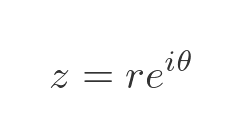The modulus of z is the distance from the origin to the point z on an Argand diagram. The argument of z is the angle z makes with the x-axis: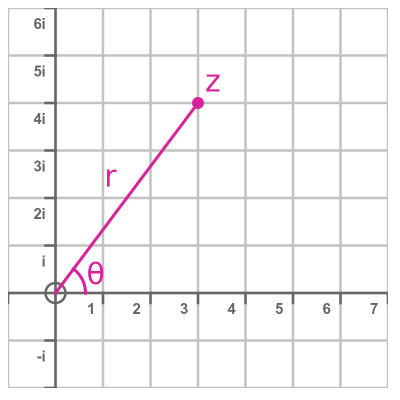When we multiply two complex numbers z1 and z2 that are expressed in this form, the normal rules of the exponential function apply: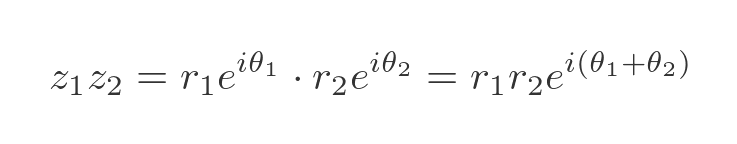We multiply the moduli r1 and r2. We add the arguments Θ1 and Θ2. That is exactly the same as we would do if the exponents were real numbers.

## The value i in modulus-argument form

We will be using i quite a lot, so it is useful to know its modulus-argument form. Here is i on an Argand diagram: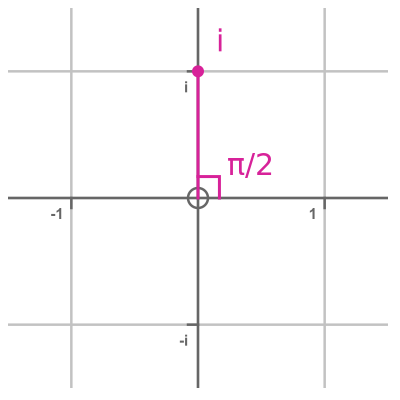i is 1 unit vertically above the origin. So the length r is 1, and the angle is π/2 radians (which is 90 degrees of course). Here is the exponential form of i: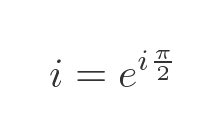If we multiply any number z by i, then in modulus-argument form this is: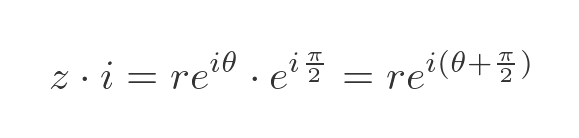In other words, multiplying by z by i simply rotates z by π/2 radians about the origin.

## Integer powers of i

Before calculating i to the power i, it is worth looking at i raised to a real power, as this will give us a couple of insights into the problem. We can calculate i squared like this: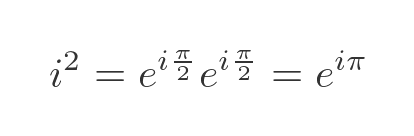This value has a unit length and an angle of π radians (half a full turn). This makes it equal to -1. But we already know that i squared is -1, by definition. So (as expected) the modulus-argument form of i squared gives the same result as simple complex number multiplication.

We can find i cubed in the same way. This time the angle is 3π/2 radians (three-quarters of a full turn), so the result is -i: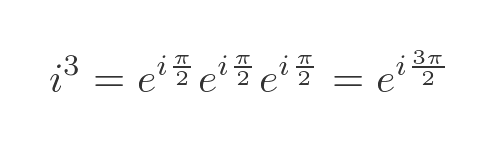i to the fourth has an angle of radians a full turn), so the result is 1: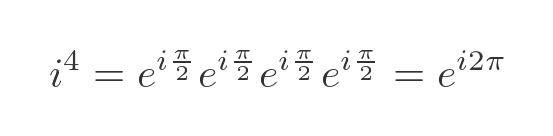Here are i and its second, third and fourth powers plotted on an Argand diagram: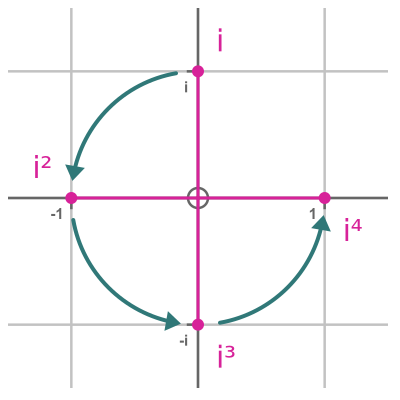It is no great surprise that i to the fourth power is 1. i to the fourth is just i squared then squared again, and since i squared is -1 then we would expect i to the fourth to be 1.

We can generalise this and say that i to any integer power is equal to: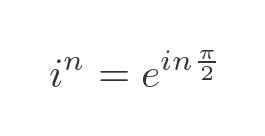Using this we can find the fifth, sixth and seventh powers on the Argand diagram: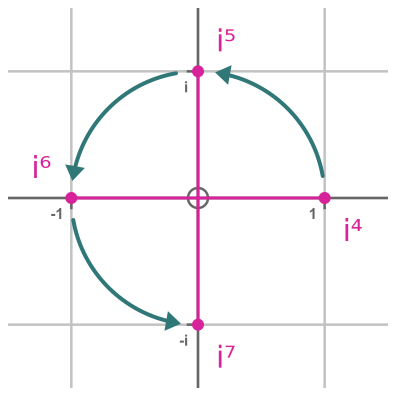Higher integer powers of i continue rotating round and round the unit circle.

## Takeaways

There are two important takeaways from this. The first is that raising i to the power n, in modulus-argument form, works in the same way as raising any other exponential to a power n. We just multiply the exponent by n: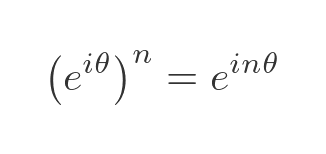The second is that there are infinitely many ways to express i in modulus argument form. Since i to the fourth is equal to 1, it follows that: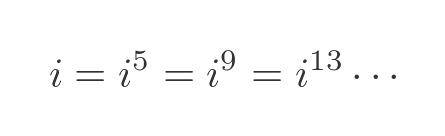In modulus-argument form: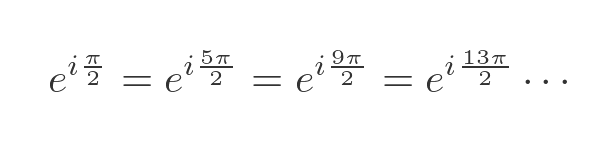In fact, for any complex number z with argument Θ, if we add an integer multiple of to Θ, we will get the same number. This follows from Euler's formula: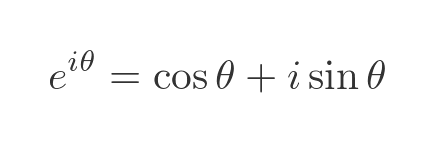Adding a multiple of to the angle does not change the value of the sine or cosine functions, because those functions are periodic with period , so: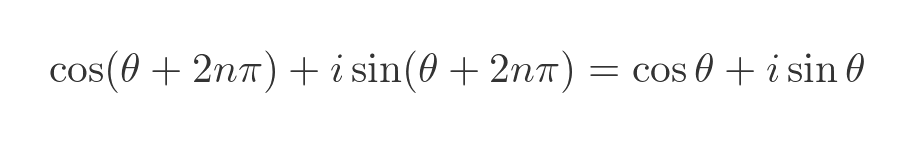## Integer roots of i

So what is the square root of i? Well, the square root of a real number x is given by raising x to the power one-half. What happens if we try the same thing with i?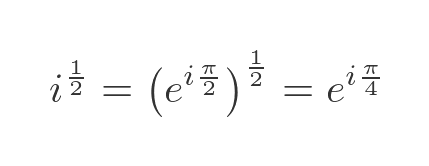But remember that i can also be written as i to the power 5. If we take the square root of this alternate form we get a second square root: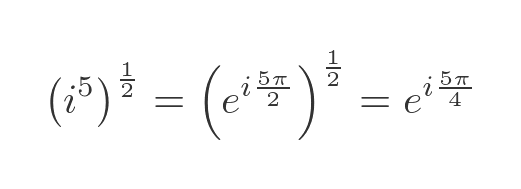We can draw these two roots on an Argand diagram: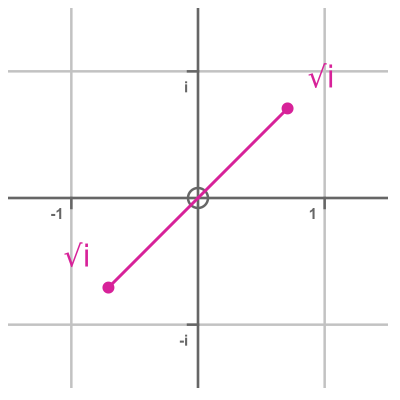We can do this again with i to the power 9 (which is also equal to i):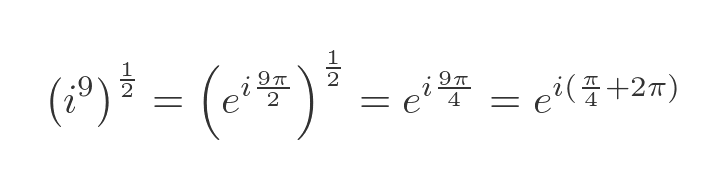This gives a result that has an argument of π/4 plus . Since adding has no effect on the value of a complex number, this result is identical to the original case where the argument was π/4. There are only two distinct square roots of i.

In fact, every complex number (except 0) has two distinct square roots, 3 distinct cube roots, and n distinct nth roots.

## Takeaway

i raised to a power p can sometimes have multiple values. Those values can be found by calculating the powers of the following equivalent numbers: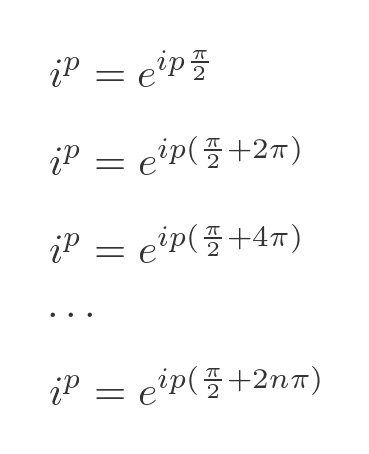Not all of these roots are necessarily distinct.

## i to the power i

So now we are in a position to calculate the value of i to the power i. We will assume that we can raise i to the power i simply by setting p to the value i in the formula above. This can be shown to be true, but we won't prove it here.

Here is the result: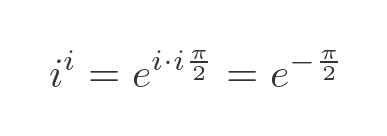This is a very interesting result. The two i terms multiply to give -1, so the exponent is now a real number. This means that the power is a real number expression!

i to the power i is simply the exponential of -π/2. Which has a real value of approximately 0.207880.

But it gets a little weirder. We also have to consider the other possible results based on the alternate modulus-argument forms of i. For example when n equals 1, we add to the exponent: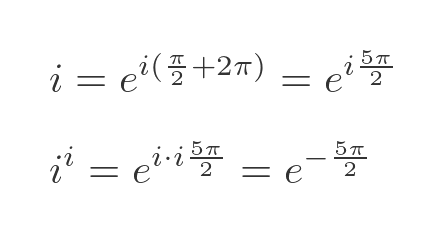This gives a value of approximately 0.000388203.

We can use negative values of n too, of course. When n equals -1, we subtract from the exponent: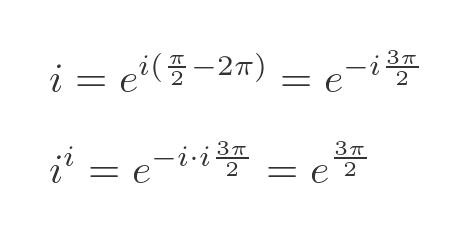This gives a value of approximately 111.318.

Since this formula is based on the exponential function of a real number, every different value of n will give a unique, real result.

So i to the power i has an infinite number of solutions, and they are all real numbers.